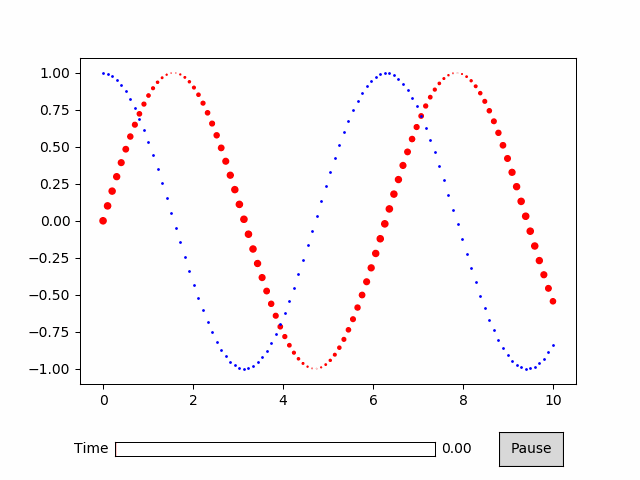# Scatter¶

:

%matplotlib qt
import numpy as np
import matplotlib as mpl
import matplotlib.pyplot as plt
import animatplot as amp


Sample data:

:

x = np.linspace(0, 10, 100)
t = np.linspace(0, 2*np.pi, 100)

X, T = np.meshgrid(x, t)

Y1 = np.sin(X+T)
Y2 = np.sin(X+T+np.pi/2)

Sizes = 20*np.cos(X-5*T)**2


Producing the animation:

:

timeline = amp.Timeline(T, fps=10)
block1 = amp.blocks.Scatter(X, Y1, Sizes, c='r')
block2 = amp.blocks.Scatter(X, Y2, 1, c='b')
anim = amp.Animation([block1, block2], timeline)

anim.controls()
anim.save_gif('scatter') #save animation for the docs
plt.show()